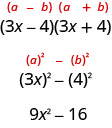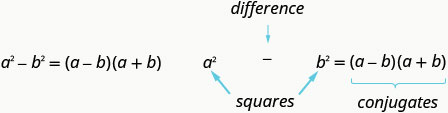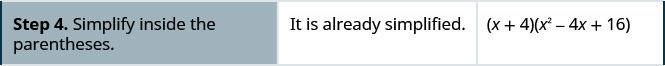# 6.4: Factor 特殊产品

•• OpenStax
• OpenStax
$$\newcommand{\vecs}{\overset { \rightharpoonup} {\mathbf{#1}} }$$ $$\newcommand{\vecd}{\overset{-\!-\!\rightharpoonup}{\vphantom{a}\smash {#1}}}$$$$\newcommand{\id}{\mathrm{id}}$$ $$\newcommand{\Span}{\mathrm{span}}$$ $$\newcommand{\kernel}{\mathrm{null}\,}$$ $$\newcommand{\range}{\mathrm{range}\,}$$ $$\newcommand{\RealPart}{\mathrm{Re}}$$ $$\newcommand{\ImaginaryPart}{\mathrm{Im}}$$ $$\newcommand{\Argument}{\mathrm{Arg}}$$ $$\newcommand{\norm}{\| #1 \|}$$ $$\newcommand{\inner}{\langle #1, #2 \rangle}$$ $$\newcommand{\Span}{\mathrm{span}}$$ $$\newcommand{\id}{\mathrm{id}}$$ $$\newcommand{\Span}{\mathrm{span}}$$ $$\newcommand{\kernel}{\mathrm{null}\,}$$ $$\newcommand{\range}{\mathrm{range}\,}$$ $$\newcommand{\RealPart}{\mathrm{Re}}$$ $$\newcommand{\ImaginaryPart}{\mathrm{Im}}$$ $$\newcommand{\Argument}{\mathrm{Arg}}$$ $$\newcommand{\norm}{\| #1 \|}$$ $$\newcommand{\inner}{\langle #1, #2 \rangle}$$ $$\newcommand{\Span}{\mathrm{span}}$$$$\newcommand{\AA}{\unicode[.8,0]{x212B}}$$

##### 学习目标

• 因子完美方形三项式
• 正方形的因子差
• 因子总和和和与立方差

1. 简化：$$(3x^2)^3$$
2. 乘以：$$(m+4)^2$$
3. 乘以：$$(x−3)(x+3)$$

## Factor Perfect Square 三项式##### 完美的方形三项式图案

$a^2+2ab+b^2=(a+b)^2\nonumber$

$a^2−2ab+b^2=(a−b)^2\nonumber$

##### 示例$$\PageIndex{1}$$: How to Factor Perfect Square Trinomials##### 示例$$\PageIndex{2}$$

$$(2x+3)^2$$

##### 示例$$\PageIndex{3}$$

$$(3y+4)^2$$

##### FACTOR 完美方形三项式

$$\begin{array} {lllll} \textbf{Step 1.} &\text{Does the trinomial fit the pattern?} &\quad &\hspace{7mm} a^2+2ab+b^2 &\hspace{7mm} a^2−2ab+b^2 \\ &\text{Are the first and last terms perfect squares?} &\quad & &\\ &\text{Write them as squares.} &\quad &\hspace{5mm}(a)^2\hspace{16mm} (b)^2 &\hspace{6mm}(a)^2\hspace{16mm} (b)^2 \\ &\text{Check the middle term. Is it }2ab? &\quad &\hspace{12mm} {\,}^{\searrow}{\,}_{2·a·b}{\,}^{\swarrow} &\hspace{12mm} {\,}^{\searrow}{\,}_{2·a·b}{\,}^{\swarrow} \\ \textbf{Step 2.} &\text{Write the square of the binomial.} &\quad &\hspace{13mm} (a+b)^2 &\hspace{13mm} (a−b)^2 \\ \textbf{Step 3.} &\text{Check by multiplying.} & & & \end{array}$$

##### 示例$$\PageIndex{4}$$

 $$81 y^{2}-72 y+16$$ 第一个和最后一个项是完美的正方形吗？查看中间术语。它匹配$$(a−b)^2$$吗？ 是的。写成二项式的平方。 $$(9 y-4)^{2}$$ 乘以检查： $(9y−4)^2\nonumber$$(9y)^2−2·9y·4+4^2\nonumber$$81y^2−72y+16\checkmark\nonumber$
##### 示例$$\PageIndex{5}$$

$$(8y−5)^2$$

##### 示例$$\PageIndex{6}$$

$$(4z−9)^2$$

##### 示例$$\PageIndex{7}$$

 $$36 x^{2}+84 x y+49 y^{2}$$ 测试每个术语以验证模式。因子。 $$(6 x+7 y)^{2}$$ 乘法检查。 $(6x+7y)^2\nonumber$$(6x)^2+2·6x·7y+(7y)^2\nonumber$$36x^2+84xy+49y^2\checkmark\nonumber$
##### 示例$$\PageIndex{8}$$

$$(7x+6y)^2$$

##### 示例$$\PageIndex{9}$$

$$(8m+7n)^2$$

##### 示例$$\PageIndex{10}$$

 $$100 x^{2} y-80 x y+16 y$$ 有全球气候基金吗？ 是的$$4y$$，所以把它排除在外。 $$4 y\left(25 x^{2}-20 x+4\right)$$ 这是一个完美的方形三项式吗？ 验证图案。因子。 $$4 y(5 x-2)^{2}$$ 记住：在最终产品中保留因子 4 y。 查看： $4y(5x−2)^2\nonumber$$4y[(5x)2−2·5x·2+22]\nonumber$ $4y(25x2−20x+4)\nonumber$100x2y−80xy+16y\ 复选标记\]
##### 示例$$\PageIndex{11}$$

$$2y(2x−3)^2$$

##### 示例$$\PageIndex{12}$$

$$3q(3p+5)^2$$

## 正方形的因子差##### 正方差图案##### 示例$$\PageIndex{13}$$: How to Factor a Trinomial Using the Difference of Squares##### 示例$$\PageIndex{14}$$

$$(11m−1)(11m+1)$$

##### 示例$$\PageIndex{15}$$

$$(9y−1)(9y+1)$$

##### 平方的因子差。

$$\begin{array} {llll} \textbf{Step 1.} &\text{Does the binomial fit the pattern?} &\qquad &\hspace{5mm} a^2−b^2 \\ &\text{Is this a difference?} &\qquad &\hspace{2mm} \text{____−____} \\ &\text{Are the first and last terms perfect squares?} & & \\ \textbf{Step 2.} &\text{Write them as squares.} &\qquad &\hspace{3mm} (a)^2−(b)^2 \\ \textbf{Step 3.} &\text{Write the product of conjugates.} &\qquad &(a−b)(a+b) \\ \textbf{Step 4.} &\text{Check by multiplying.} & & \end{array}$$

##### 示例$$\PageIndex{16}$$

$$\begin{array} {lll} &\quad &144x^2−49y^2 \\ \text{Is this a difference of squares? Yes.} &\quad &(12x)^2−(7y)^2 \\ \text{Factor as the product of conjugates.} &\quad &(12x−7y)(12x+7y) \\ \text{Check by multiplying.} &\quad &(12x−7y)(12x+7y) \\ \text{Check by multiplying.} &\quad & \\ &\quad & \\ &\quad & \\ \hspace{14mm} (12x−7y)(12x+7y) &\quad & \\ \hspace{21mm} 144x^2−49y^2\checkmark &\quad & \end{array}$$

##### 示例$$\PageIndex{17}$$

$$(14m−5n)(14m+5n)$$

##### 示例$$\PageIndex{18}$$

$$(11p−3q)(11p+3q)$$

##### 示例$$\PageIndex{19}$$

$$\begin{array} {ll} &48x^4y^2−243y^2 \\ \text{Is there a GCF? Yes, }3y^2\text{—factor it out!} &3y^2(16x^4−81) \\ \text{Is the binomial a difference of squares? Yes.} &3y^2\left((4x^2)^2−(9)^2\right) \\ \text{Factor as a product of conjugates.} &3y^2(4x^2−9)(4x^2+9) \\ \text{Notice the first binomial is also a difference of squares!} &3y^2((2x)^2−(3)^2)(4x^2+9) \\ \text{Factor it as the product of conjugates.} &3y^2(2x−3)(2x+3)(4x^2+9) \end{array}$$

$$\begin{array} {l} \text{Check by multiplying:} \\ \hspace{10mm} 3y^2(2x−3)(2x+3)(4x^2+9) \\ \\ \\ \hspace{15mm} 3y^2(4x^2−9)(4x^2+9) \\ \hspace{20mm} 3y^2(16x^4−81) \\ \hspace{19mm} 48x^4y^2−243y^2\checkmark\end{array}$$

##### 示例$$\PageIndex{20}$$

$$2y^2(x−2)(x+2)(x^2+4)$$

##### 示例$$\PageIndex{21}$$

$$7c^2(a−b)(a+b)(a^2+b^2)$$

##### 示例$$\PageIndex{22}$$

 $$x^{2}-6 x+9-y^{2}$$ 通过对前三个项进行分组来进行分数。 $$\underbrace{x^{2}-6 x+9} - y^{2}$$ 使用完美的方形三项式图案。 $$(x-3)^{2}-y^{2}$$ 这是正方差吗？ 是的。 是的——把它们写成正方形。因子是共轭物的乘积。$$(x-3-y)(x-3+y)$$

##### 示例$$\PageIndex{23}$$

$$(x−5−y)(x−5+y)$$

##### 示例$$\PageIndex{24}$$

$$(x+3−2y)(x+3+2y)$$

## 多维数据集的因子和和和差

$a^3+b^3=(a+b)(a^2−ab+b^2\nonumber$

$a^3−b^3=(a−b)(a^2+ab+b^2)\nonumber$

 $$\color{red}(a+b) \color{black} \left(a^{2}-a b+b^{2}\right)$$ 分发。 $$\color{red}a \color{black}\left(a^{2}-a b+b^{2}\right)+ \color{red}b \color{black}\left(a^{2}-a b+b^{2}\right)$$ 乘以。 $$a^{3}-a^{2} b+a b^{2}+a^{2} b-a b^{2}+b^{3}$$ 将相似的术语组合在一起。 $$a^{3}+b^{3}$$
##### 多维数据集的总和与差值模式

$a^3+b^3=(a+b)(a^2−ab+b^2\nonumber$$a^3−b^3=(a−b)(a^2+ab+b^2)\nonumber$n 1 2 3 4 5 6 7 8 9 10
$$n^3$$ 1 8 27 64 125 216 343 512 729 1000
##### 示例$$\PageIndex{25}$$: How to Factor the Sum or Difference of Cubes##### 示例$$\PageIndex{26}$$

$$(x+3)(x^2−3x+9)$$

##### 示例$$\PageIndex{27}$$

$$(y+2)(y^2−2y+4)$$

##### 分解立方体的总和或差值。
1. 二项式是否符合立方体模式的总和或差异？
是总和还是差异？
第一个和最后一个学期是完美的立方体吗？
2. 把它们写成立方体。
3. 使用立方体的总和或差值模式。
4. 在圆括号内简化。
5. 通过乘以因子进行检查。
##### 示例$$\PageIndex{28}$$

 $$27 u^{3}-125 v^{3}$$ 这个二项式是不同的。 第一个和最后一个 术语是完美的立方体。 将术语写成立方体。使用立方体差异图案。简化。乘法检查。 我们会把支票留给你。
##### 示例$$\PageIndex{29}$$

$$(2x−3y)(4x^2+6xy+9y^2)$$

##### 示例$$\PageIndex{30}$$

$$(10m−5n)(100m^2+50mn+25n^2)$$

##### 示例$$\PageIndex{31}$$

 $$6 x^{3} y+48 y^{4}$$ 将共同因素考虑在内。 $$6 y\left(x^{3}+8 y^{3}\right)$$ 这个二项式是一个总和第一个和最后一个 项是完美的立方体。 将术语写成立方体。使用立方体总和模式。简化。##### 示例$$\PageIndex{32}$$

$$4(5p+q)(25p^2−5pq+q^2)$$

##### 示例$$\PageIndex{33}$$

$$2(6c+7d)(36c^2−42cd+49d^2)$$

##### 示例$$\PageIndex{34}$$

 $$(x+5)^{3}-64 x^{3}$$ 这个二项式是不同的。 第一个和 最后一个术语是完美的立方体。 将术语写成立方体。使用立方体差异图案。简化。 $$(x+5-4 x)\left(x^{2}+10 x+25+4 x^{2}+20 x+16 x^{2}\right)$$ $$(-3 x+5)\left(21 x^{2}+30 x+25\right)$$ 乘法检查。 我们会把支票留给你。
##### 示例$$\PageIndex{35}$$

$$(−2y+1)(13y^2+5y+1)$$

##### 示例$$\PageIndex{36}$$

$$(−4n+3)(31n^2+21n+9)$$

## 关键概念

• 完美方形三项式图案：如果 ab 是实数，

$\begin{array} {l} a^2+2ab+b^2=(a+b)^2 \\ a^2−2ab+b^2=(a−b)^2\end{array} \nonumber$

• 如何分解完美的正方三项式。
$$\begin{array} {lllll} \textbf{Step 1.} &\text{Does the trinomial fit the pattern?} &\quad &\hspace{7mm} a^2+2ab+b^2 &\hspace{7mm} a^2−2ab+b^2 \\ &\text{Are the first and last terms perfect squares?} &\quad & &\\ &\text{Write them as squares.} &\quad &\hspace{5mm}(a)^2\hspace{16mm} (b)^2 &\hspace{6mm}(a)^2\hspace{16mm} (b)^2 \\ &\text{Check the middle term. Is it }2ab? &\quad &\hspace{12mm} {\,}^{\searrow}{\,}_{2·a·b}{\,}^{\swarrow} &\hspace{12mm} {\,}^{\searrow}{\,}_{2·a·b}{\,}^{\swarrow} \\ \textbf{Step 2.} &\text{Write the square of the binomial.} &\quad &\hspace{13mm} (a+b)^2 &\hspace{13mm} (a−b)^2 \\ \textbf{Step 3.} &\text{Check by multiplying.} & & & \end{array}$$
• 方差模式：如果 a、ba、b 是实数，• 如何分解平方差。
$$\begin{array} {llll} \textbf{Step 1.} &\text{Does the binomial fit the pattern?} &\qquad &\hspace{5mm} a^2−b^2 \\ &\text{Is this a difference?} &\qquad &\hspace{2mm} \text{____−____} \\ &\text{Are the first and last terms perfect squares?} & & \\ \textbf{Step 2.} &\text{Write them as squares.} &\qquad &\hspace{3mm} (a)^2−(b)^2 \\ \textbf{Step 3.} &\text{Write the product of conjugates.} &\qquad &(a−b)(a+b) \\ \textbf{Step 4.} &\text{Check by multiplying.} & & \end{array}$$
• 多维数据集的总和与差值模式
$$\begin{array} {l} a^3+b3=(a+b)(a^2−ab+b^2) \\ a^3−b^3=(a−b)(a^2+ab+b^2) \end{array}$$
• 如何分解立方体的总和或差值。
1. 二项式是否符合立方体模式的总和或差异？
是总和还是差异？
第一个和最后一个学期是完美的立方体吗？
2. 把它们写成立方体。
3. 使用立方体的总和或差值模式。
4. 在圆括号内简化
5. 通过乘以因子进行检查。